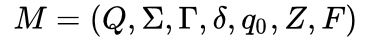## An example of a deterministic finite automaton that accepts only binary numbers that are multiples of 3. The state q0 is both the start state and an accept state. For example, the string "1001" leads to the state sequence q0, q1, q2, q1, q0, and is hence accepted.Please Follow the Video and Then go for the Code for Better Understanding.

``````
dfa={0:{'0':0,'1':1},
1:{'0':2,'1':0},
2:{'0':1,'1':2}}

def accepts(delta,start,final,sigma):
state=start
for input in sigma:
state=dfa[state][input]
return state in final
print(accepts(dfa,0,{0},'1001'))
``````
``` ```

# Nondeterministic finite automaton:

## To perform this Operation Please install this module just type in CMD: pip install automata-lib

``````
from automata.fa.nfa import NFA
nfa = NFA(
states={'q0', 'q1', 'q2'},
input_symbols={'1', '0'},
transitions={
'q0': {'1': {'q1'}},
# Use '' as the key name for empty string (lambda/epsilon) transitions
'q1': {'1': {'q1'}, '': {'q2'}},
'q2': {'0': {'q0'}}
},
initial_state='q0',
final_states={'q1'}
)
``````
``` ```

# PushDown Automaton:

## A PDA is formally defined as a 7-tuple:where

## Example:DPDA which which matches zero or more '0's, followed by the same number of '1's (accepting by final state)

``````
from automata.pda.dpda import DPDA
dpda = DPDA(
states={'q0', 'q1', 'q2', 'q3'},
input_symbols={'0', '1'},
stack_symbols={'0', '1'},
transitions={
'q0': {
'0': {'0': ('q1', ('1', '0'))}  # transition pushes '1' to stack
},
'q1': {
'0': {'1': ('q1', ('1', '1'))},
'1': {'1': ('q2', '')}  # transition pops from stack
},
'q2': {
'1': {'1': ('q2', '')},
'': {'0': ('q3', ('0',))}  # transition does not change stack
}
},
initial_state='q0',
initial_stack_symbol='0',
final_states={'q3'},
acceptance_mode='final_state'
)
```
```

# Turing Machine:

## Example:DTM which matches all strings beginning with '0's, and followed by the same number of '1's

``````
from automata.tm.dtm import DTM
dtm = DTM(
states={'q0', 'q1', 'q2', 'q3', 'q4'},
input_symbols={'0', '1'},
tape_symbols={'0', '1', 'x', 'y', '.'},
transitions={
'q0': {
'0': ('q1', 'x', 'R'),
'y': ('q3', 'y', 'R')
},
'q1': {
'0': ('q1', '0', 'R'),
'1': ('q2', 'y', 'L'),
'y': ('q1', 'y', 'R')
},
'q2': {
'0': ('q2', '0', 'L'),
'x': ('q0', 'x', 'R'),
'y': ('q2', 'y', 'L')
},
'q3': {
'y': ('q3', 'y', 'R'),
'.': ('q4', '.', 'R')
}
},
initial_state='q0',
blank_symbol='.',
final_states={'q4'}
)

``````

# DFA Semi Automated One-Click Place Bulk Orders Utilizing Upstox Trade Button Via Auto Populated Upstox Excel/CSV File:

## Upstox Provides Trade Button from that we are able to trade our orders in Bulk with the help of Excel/CSV just follow the Code Input and upload your stock with right quantity and input to avail this offer you need to be upstox users and have an account under my referral code by clicking the link https://upstox.com/open-account/?f=36K0 SignUp with Email Address,Mobile Number. Then for SignIn Enter PAN Details,DOB and for UCC just add 168010. After your account is activated you will be registered to use this tool for free.Placing Orders as per DFA Please Check the Video and Follow the Proper Instructions:

``````
import pandas as pd
import csv
dfa={0:{'0':0,'1':1},
1:{'0':2,'1':0},
2:{'0':1,'1':2}}
exchange='NSE'
quantity=1
signal='01010101'
columns=[ 'symbol', 'exchange', 'series', 'quantity','price', 'side',
'complexity', 'orderType','stoploss','target','trailingTicks','triggerPrice', 'position', 'tif']

with open('upx_excel.csv','w') as csvfile:
wr=csv.writer(csvfile)
wr.writerow(columns)
symbol='RELIANCE'
exchange='NSE'
series='EQ'
quantity=1
complexity='SIMPLE'
orderType='l'
position='i'
tif='day'
stoploss='0'
target='0'
trailingTicks='0'
triggerPrice='0'
price=1987

def accepts(delta,start,final,sigma):
state=start
for input in sigma:
state=dfa[state][input]
return state in final
print(accepts(dfa,0,{1},signal))
if(accepts(dfa,0,{1},signal)==True):
print(len(signal))
for i in range(len(signal)):
print()
if(signal[i]=='1'):
with open('upx_excel.csv','a') as csvfile:
wr=csv.writer(csvfile)
tif])
if(signal[i]=='0'):
print(signal[i],'SELL')
with open('upx_excel.csv','a') as csvfile:
wr=csv.writer(csvfile)
wr.writerow([symbol,exchange,series,quantity,'{}'.format( price-i),'SELL',complexity,orderType,stoploss,target,trailingTicks,triggerPrice,position,
tif
])

```
```